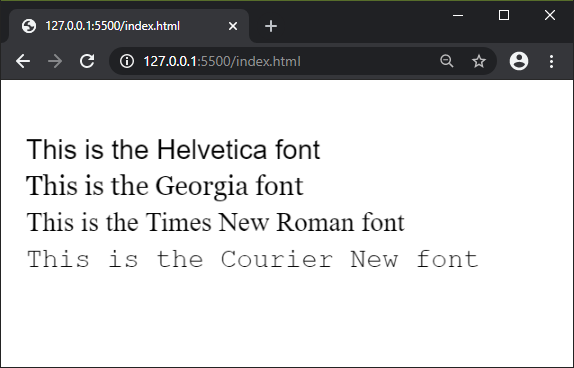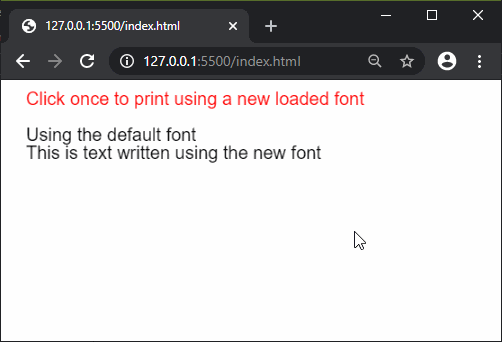# p5.js | textFont() Function

• Last Updated : 16 Apr, 2020

The textFont() function in p5.js is used to specify the font that will be used to draw text using the text() function. In the WEBGL mode, only the fonts loaded by the loadFont() method are supported.

Syntax:

`textFont( font, size )`

Parameters: This function accepts two parameters as mentioned above and described below:

• font: It is a string that specifies the name of the web safe font or a font object loaded by the loadFont() function.
• size: It is a number that specifies the size of the font to use. It is an optional parameter.

Return Value: It is an object that contains the current font.

Below examples illustrate the textFont() function in p5.js:

Example 1: This example shows the use of web safe fonts that are generally available on all systems.

 `function` `setup() {``  ``createCanvas(600, 300);``  ``textSize(30);`` ` `  ``textFont(``'Helvetica'``);``  ``text(``'This is the Helvetica font'``, 20, 80);``  ``textFont(``'Georgia'``);``  ``text(``'This is the Georgia font'``, 20, 120);``  ``textFont(``'Times New Roman'``);``  ``text(``'This is the Times New Roman font'``, 20, 160);``  ``textFont(``'Courier New'``);``  ``text(``'This is the Courier New font'``, 20, 200);``}`

Output:Example 2: This example shows the use of a font loaded using the loadFont() function.

 `let newFont;`` ` `function` `preload() {``  ``newFont = loadFont(``'fonts/Montserrat.otf'``);``}`` ` `function` `setup() {``  ``createCanvas(400, 200);``  ``textSize(20);``  ``fill(``"red"``);``  ``text(``'Click once to print using "``    ``+ "a new loaded font'``, 20, 20);``  ``fill(``"black"``);`` ` `  ``text(``'Using the default font'``, 20, 60);``  ``text(``'This is text written using"``        ``+ " the new font'``, 20, 80);``}`` ` `function` `mouseClicked() {``  ``textFont(newFont);``  ``textSize(20);``  ``text(``'Using the Montserrat font'``, 20, 140);``  ``text(``'This is text written using the"``       ``+ " new loaded font'``, 20, 160);``}`

Output:Online editor: https://editor.p5js.org/

Reference: https://p5js.org/reference/#/p5/textFont

My Personal Notes arrow_drop_up